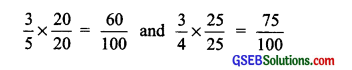# GSEB Solutions Class 8 Maths Chapter 1 Rational Numbers Ex 1.2

Gujarat Board GSEB Textbook Solutions Class 8 Maths Chapter 1 Rational Numbers Ex 1.2 Textbook Questions and Answers.

## Gujarat Board Textbook Solutions Class 8 Maths Chapter 1 Rational Numbers Ex 1.2

Question 1.
Represent these numbers on the number line.

1. $$\frac { 7 }{ 4 }$$
2. $$\frac { -5 }{ 6 }$$

Solution:
(i) To represent $$\frac { 7 }{ 4 }$$ we make 7 markings each at a distance equal to $$\frac { 1 }{ 4 }$$ on the right of 0.

The 7th point represents the rational number $$\frac { 7 }{ 4 }$$ as shown in the figure.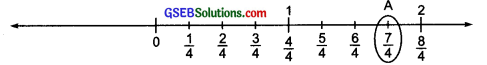The point A $$\frac { 7 }{ 4 }$$.

(ii) To represent ($$\frac { 7 }{ 4 }$$) on the nmber Line, we make 5 each at a distance equal to $$\frac { 1 }{ 6 }$$ on the left of 0. We consider the 5th point as shwn in the figure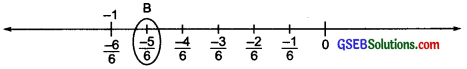The point B represent ($$\frac { -5 }{ 6 }$$)Question 2.
Represent $$\frac { -2 }{ 11 }$$, $$\frac { -5 }{ 11 }$$, $$\frac { -9 }{ 11 }$$. on the number line.
Solution:
TO represent $$\frac { -2 }{ 11 }$$, $$\frac { -5 }{ 11 }$$ and $$\frac { -9 }{ 11 }$$ on a number line, we make 11 marking each being equal to distance $$\frac { 1 }{ 11 }$$ on the left of 0.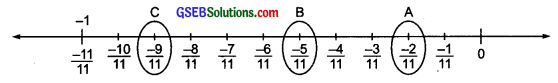• The point A represent $$\frac { -2 }{ 11 }$$
• The point B represent $$\frac { -5 }{ 11 }$$
• The point C represent $$\frac { -9 }{ 11 }$$

Question 3.
Write five rational numbers which are smaller than 2.
Solution:
There can be unlimited rational numbers smaller than 2. Five of them are:
0, -1, $$\frac { 1 }{ 2 }$$, $$\frac { 1 }{ 2 }$$, 1Question 4.
Find ten rational numbers between $$\frac { -2 }{ 5 }$$ and $$\frac { 1 }{ 2 }$$
Solution:
To convert $$\frac { -2 }{ 5 }$$ and $$\frac { 1 }{ 2 }$$ having the same denominators:
We have $$\frac { -2 }{ 5 }$$ = $$\frac{-2 \times 4}{5 \times 4}$$ = $$\frac { -8 }{ 20 }$$ and $$\frac { 1 }{ 2 }$$ = $$\frac{1 \times 10}{2 \times 10}$$ = $$\frac { 10 }{ 20 }$$
∵ The rational numbers between $$\frac { 10 }{ 20 }$$ and $$\frac { -8 }{ 20 }$$ are $$\frac { 9 }{ 20 }$$, $$\frac { 8 }{ 20 }$$, $$\frac { 7 }{ 20 }$$, $$\frac { 6 }{ 20 }$$, …., $$\frac { -6 }{ 20 }$$ , $$\frac { -7 }{ 20 }$$
We can take any 10 of them.
∵ ten rational nnumbers between $$\frac { -2 }{ 5 }$$ and $$\frac { 1 }{ 2 }$$ are:

(i) $$\frac { 9 }{ 20 }$$
(ii) $$\frac { 8 }{ 20 }$$
(iii) $$\frac { 7 }{ 20 }$$
(iv) $$\frac { 6 }{ 20 }$$
(v) $$\frac { 5 }{ 20 }$$
(vi) $$\frac { 4 }{ 20 }$$
(vii) $$\frac { 3 }{ 20 }$$
(viii) $$\frac { 2 }{ 20 }$$
(ix) $$\frac { 1 }{ 20 }$$
(x) 0

Question 5.
Find five rational numbers between:

1. $$\frac { 2 }{ 3 }$$ and $$\frac { 4 }{ 5 }$$
2. $$\frac { -3 }{ 2 }$$ and $$\frac { 5 }{ 3 }$$
3. $$\frac { 1 }{ 4 }$$ and $$\frac { 1 }{ 2 }$$

solution:
(i) Coverting $$\frac { 2 }{ 3 }$$ and $$\frac { 4 }{ 5 }$$ having same denominators such that difference between the numerators is more than 5.
We have $$\frac { 2 }{ 3 }$$ = $$\frac{2 \times 20}{3 \times 20}$$ = $$\frac { 40 }{ 60 }$$ and $$\frac { 4 }{ 5 }$$ = $$\frac{4 \times 12}{5 \times 12}$$ = $$\frac { 48 }{ 60 }$$
Now, any five rational number between
$$\frac{40}{60}\left(=\frac{2}{3}\right)$$ and $$\frac{48}{60}\left(=\frac{4}{5}\right)$$ are: $$\frac { 41 }{ 60 }$$, $$\frac { 42 }{ 60 }$$, $$\frac { 43 }{ 60 }$$ , $$\frac { 44 }{ 60 }$$, $$\frac { 45 }{ 60 }$$

(ii) Converting $$\frac { -3 }{ 2 }$$ and $$\frac { 5 }{ 3 }$$ with same denominators, we have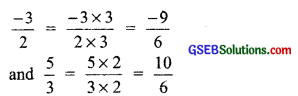∴ Five rational numbers between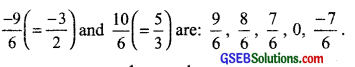(iii) Converting $$\frac { 1 }{ 4 }$$ and $$\frac { 1 }{ 2 }$$ to rational numbers with the same denominators, we have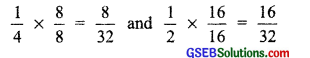∴ Five rational numbers between $$\frac { 1 }{ 4 }$$ and $$\frac { 1 }{ 2 }$$ i.e., $$\frac { 8 }{ 32 }$$ and $$\frac { 16 }{ 32 }$$ are: $$\frac { 9 }{ 32 }$$, $$\frac { 10 }{ 32 }$$, $$\frac { 11 }{ 32 }$$, $$\frac { 12 }{ 32 }$$, $$\frac { 13 }{ 32 }$$Question 6.
Write five rational numbers greater than – 2.
Solution:
Five rational numbers greater than – 2 are: $$\frac { -3 }{ 2 }$$, -1, $$\frac { -1 }{ 2 }$$, 0, $$\frac { 1 }{ 2 }$$
Note: There ten rationalnumbers greater than – 2

Question 7.
Find the rational numbers between $$\frac { 3 }{ 5 }$$ and $$\frac { 3 }{ 4 }$$.
Solution:
Converting $$\frac { 3 }{ 5 }$$ and $$\frac { 3 }{ 4 }$$ such that they between $$\frac { 3 }{ 4 }$$ and $$\frac { 3 }{ 4 }$$ such that they have common denominators and their numerators have difference of more than 10, i.each